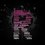# $3=1$? Please inform if there is any mistake or any contradiction in this

\begin{aligned} 3\sin^{-1} x &=& 2\sin^{-1} x + \sin^{-1} x \\ &=& \sin^{-1} (2x \sqrt{1-x^2} ) + \sin^{-1} x \\ &=& \sin^{-1} \left( 2x \sqrt{1-x^2} \sqrt{1-x^2} + x \sqrt{1 - (2x\sqrt{1-x^2} )^2} \right) \\ &=& \sin^{-1} \left( 2x(1-x^2) + x \sqrt{1 - 4x^2(1-x^2)} \right) \\ &=& \sin^{-1} \left( 2x(1-x^2) + x \sqrt{1 - 4x^2 + 4x^4)} \right) \\ &=& \sin^{-1} \left( 2x - 2x^3 + x \sqrt{(2x^2-1)^2} \right) \\ &=& \sin^{-1} (2x - 2x^3 + x(2x^2 - 1)) \\ &=& \sin^{-1} (2x - \cancel{2x^3} + \cancel{2x^3} - x) \\ &=& \sin^{-1} x \end{aligned}

Thus $3\sin^{-1} x = \sin^{-1} x$ or $3=1$?Note by Darvin Rio
5 years, 5 months ago

This discussion board is a place to discuss our Daily Challenges and the math and science related to those challenges. Explanations are more than just a solution — they should explain the steps and thinking strategies that you used to obtain the solution. Comments should further the discussion of math and science.

When posting on Brilliant:

• Use the emojis to react to an explanation, whether you're congratulating a job well done , or just really confused .
• Ask specific questions about the challenge or the steps in somebody's explanation. Well-posed questions can add a lot to the discussion, but posting "I don't understand!" doesn't help anyone.
• Try to contribute something new to the discussion, whether it is an extension, generalization or other idea related to the challenge.

MarkdownAppears as
*italics* or _italics_ italics
**bold** or __bold__ bold
- bulleted- list
• bulleted
• list
1. numbered2. list
1. numbered
2. list
Note: you must add a full line of space before and after lists for them to show up correctly
paragraph 1paragraph 2

paragraph 1

paragraph 2

[example link](https://brilliant.org)example link
> This is a quote
This is a quote
    # I indented these lines
# 4 spaces, and now they show
# up as a code block.

print "hello world"
# I indented these lines
# 4 spaces, and now they show
# up as a code block.

print "hello world"
MathAppears as
Remember to wrap math in $$ ... $$ or $ ... $ to ensure proper formatting.
2 \times 3 $2 \times 3$
2^{34} $2^{34}$
a_{i-1} $a_{i-1}$
\frac{2}{3} $\frac{2}{3}$
\sqrt{2} $\sqrt{2}$
\sum_{i=1}^3 $\sum_{i=1}^3$
\sin \theta $\sin \theta$
\boxed{123} $\boxed{123}$

Sort by:

You're making two different mistakes.

The first one is that if $x^2 \leq \frac{1}{2}$, then $\sqrt{(2x^2 -1)^2} = (1 - 2x^2) \not = (2x^2 -1)$

The second one is that if $x^2 > \frac{1}{2}$ then $|\sin^{-1}(x)| > \frac{\pi}{4}$. and $2|\sin^{-1}(x)| \geq \frac{\pi}{2}$

Therefore it should be obvious that, $2|\sin^{-1}(x)| \not = |\sin^{-1}(2x\sqrt{1-x^2})|$, since the LHS $> \frac{\pi}{2}$ and the RHS $\leq \frac{\pi}{2}$ (Range of $sin^{-1}$ is $[\frac{\pi}{2},-\frac{\pi}{2}]$)

- 5 years, 5 months ago

Isn't $3\sin^{-1} x=\sin^{-1} x$ satisfied only when $\sin^{-1}x=0$. If that is true then recalling basic identities of equations: $ac=ad\Rightarrow c=d$ (Only when a is non zero).
However in our case c=3, d=1 while a=$\sin^{-1} x$ which is 0!! Hence $3\sin^{-1} x = \sin^{-1} x \not\Rightarrow 3=1$

- 5 years, 5 months ago

i tried deriving a standard derivation......so universally doesnt it mean that sin inverse x universally is 0!!!!

- 5 years, 5 months ago

I think that there is another mistake because if everything is correct till the last step then, $2 \sin^{-1}{x}+\sin^{-1}{x}=\sin^{-1}{x}$ or $\sin^{-1}{x}=0$.

- 5 years, 5 months ago

Exactly $\sin^{-1} x=0$ that's why in last step we cannot cancel $\sin^{-1} x$ from both sides to claim $1=3$.

- 5 years, 5 months ago

The problem is that the first four lines are only valid if x=0. If not $sin^{-1} x$ would be 0 for al x, by this argumentation, but the first 4 lines make implicit assumtions that x lies in certain ranges and the intersection of those is $\{0\}$

- 5 years, 5 months ago